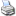# Conversions

All conversions are approximations. If in doubt, try going to Google and type in what you want to convert, for example, ‘400 degrees F in C’ or ‘1 cup in ml’. Scroll to the bottom for American English to British English foodie translations.

Temperature

275°F = 140°C = gas mark 1 = very cool
300°F = 150°C = gas mark 2 = cool
325°F = 160°C = gas mark 3 = warm
350°F = 180°C = gas mark 4 = moderate
375°F = 190°C = gas mark 5 = fairly hot
400°F = 200°C = gas mark 6 = fairly hot
425°F = 220°C = gas mark 7 = hot
450°F = 230°C = gas mark 8 = very hot
475°F = 240°C = gas mark 9 = very hot

Weight (metric to US)

30 g = 1 oz
60 g = 2 oz
90 g = 3 oz
110 g = 4 oz
140 g = 5 oz
170 g = 6 oz
200 g = 7 oz
225 g = 8 oz
250 g = 9 oz
280 g = 10 oz
310 g = 11 oz
340 g = 12 oz
370 g = 13 oz
400 g = 14 oz
425 g = 15 oz
450 g = 16 oz = 1 lb
500 g = 18 oz
570 g = 1 1/4 lb
680 g = 1 1/2 lb
900 g = 2 lb
1 kg = 2 1/4 lb
1.1 kg = 2 1/2 lb
1.4 kg = 3 lb
1.5 kg = 3 lb 5 oz
1.8 kg = 4 lb
2 kg = 4 1/2 lb
2.2 kg = 5 lb

Volume (metric to US)

60 ml = 1/4 cup
80 ml = 1/3 cup
120 ml = 1/2 cup
180 ml = 3/4 cup
240 ml = 1 cup

5 ml = 1 teaspoon
10 ml = 1 dessertspoon
15 ml = 1 tablespoon = 3 teaspoons
30 ml = 1 fl oz
60 ml = 2 fl oz
90 ml = 3 fl oz
100 ml = 3 1/2 fl oz
125 ml = 4 fl oz
150 ml = 5 fl oz
175 ml = 6 fl oz
200 ml = 7 fl oz
250 ml = 8 fl oz = 1/2 pint
270 ml = 9 fl oz
300 ml = 10 fl oz
325 ml = 11 fl oz
350 ml = 12 fl oz
400 ml = 14 fl oz
450 ml = 15 fl oz
475 ml = 16 fl oz = 1 pint
500 ml = 17 fl oz
600 ml = 20 fl oz = 1 1/4 pints
700 ml = 24 fl oz = 1 1/2 pints
800 ml = 28 fl oz = 1 3/4 pints
1 litre = 34 fl oz = approx. 2 pints

Butter or margarine

4 sticks = 1 lb = 16 oz = 450 g = 2 cups
1 stick = 1/4 lb = 4 oz = 110 g = 1/2 cup = 8 tablespoons
8 oz butter = 225 g = 1 cup
4 oz butter = 110 g = 1/2 cup
2 tablespoons butter = 1 oz = 30 g
1 tablespoon butter = 1/2 oz = 15 g

All-purpose flour and confectioners’ sugar (icing or powdered sugar)

30 g = 1/4 cup
40 g = 1/3 cup
60 g = 1/2 cup
90 g = 3/4 cup
120 g = 1 cup

Granulated, superfine or caster sugar

50 g = 1/4 cup
75 g = 1/3 cup
100 g = 1/2 cup
150 g = 3/4 cup
200 g = 1 cup

Light, golden or dark brown sugar

40 g = 1/4 cup
60 g = 1/3 cup
85 g = 1/2 cup
120 g = 3/4 cup
170 g = 1 cup

Other

Almonds (flaked): 100 g = 1 cup
Almonds (ground): 100 g = 1 cup
Apricots (dried): 200 g = 1 cup
Arborio rice: 180 g = 1 cup
Barley (pearl barley): 200 g = 1 cup
Basil (packed): 30 g = 1 cup
Basmati rice: 180 g = 1 cup
Black beans (dried): 200 g = 1 cup
Blueberries: 160 g = 1 cup
Breadcrumbs: 100 g = 1 cup
Brown rice: 180 g = 1 cup
Bulgur: 225 g = 1 cup
Cannellini beans (dried): 200 g = 1 cup
Cheddar cheese (grated): 100 g = 1 cup
Chickpeas (dried): 180 g = 1 cup
Chocolate chips: 200 g = 1 cup
Cilantro/coriander (packed): 30 g = 1 cup
Cocoa: 120 g = 1 cup
Coconut (shredded): 90 g = 1 cup
Corn (frozen): 140 g = 1 cup
Cornmeal (polenta): 225 g = 1 cup
Couscous: 170 g = 1 cup
Cranberries (dried): 120 g = 1 cup
Crystallized ginger: 140 g = 1 cup
Figs (dried): 200 g = 1 cup
Hazelnuts: 150 g = 1 cup
Mozzarella (shredded): 110 g = 1 cup
Oats (porridge oats): 80 g = 1 cup
Parmesan (grated): 100 g = 1 cup
Peas (frozen) 140 g = 1 cup
Pine nuts: 140 g = 1 cup
Prunes (dried): 200 g = 1 cup
Quinoa: 170 g = 1 cup
Raisins: 160 g = 1 cup
Split peas: 200 g = 1 cup
Walnuts: 100 g = 1 cup
Wheat germ: 110 g = 1 cup
Wholemeal flour: 160 g = 1 cup

American English to British English translations

arugula = rocket
cilantro = coriander
confectioners sugar/powdered sugar = icing sugar
cornstarch = cornflour
eggplant = aubergine
half and half = half cream, half milk
heavy cream = double cream
rutabaga = swede
Swiss cheese = Emmental
zucchini = courgetteEmail this pagePrint this page